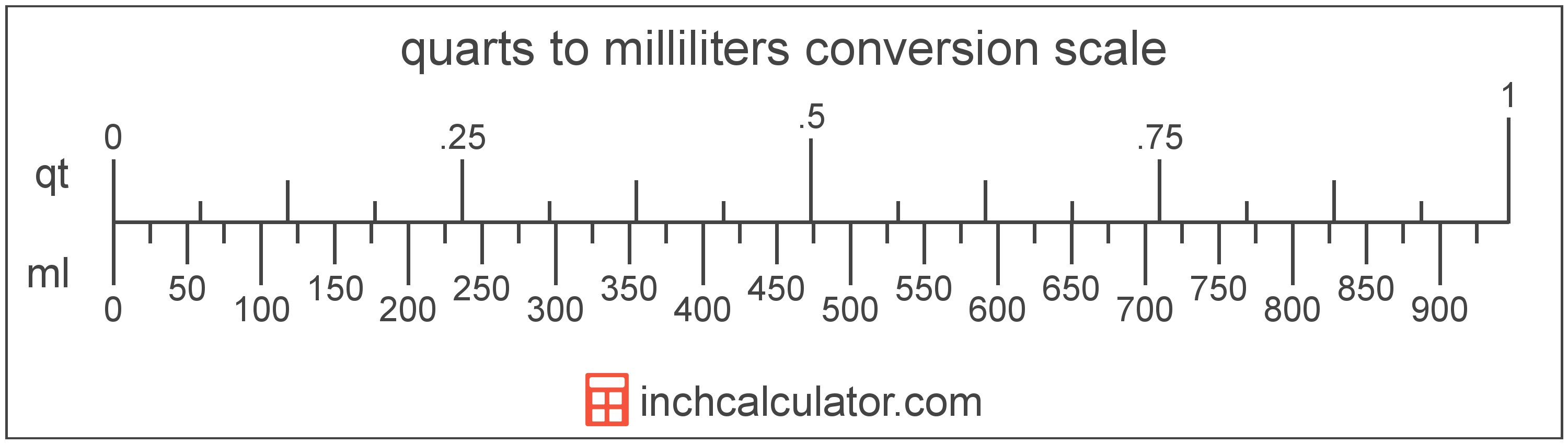# Quarts to Milliliters Converter

Enter the volume in quarts below to get the value converted to milliliters.

Results in Milliliters:1 qt = 946.352946 mL

Do you want to convert milliliters to quarts?

## How to Convert Quarts to Milliliters

To convert a measurement in quarts to a measurement in milliliters, multiply the volume by the following conversion ratio: 946.352946 milliliters/quart.

Since one quart is equal to 946.352946 milliliters, you can use this simple formula to convert:

milliliters = quarts × 946.352946

The volume in milliliters is equal to the volume in quarts multiplied by 946.352946.

For example, here's how to convert 5 quarts to milliliters using the formula above.
milliliters = (5 qt × 946.352946) = 4,731.76473 mL### How Many Milliliters Are in a Quart?

There are 946.352946 milliliters in a quart, which is why we use this value in the formula above.

1 qt = 946.352946 mL

## What is a Quart?

The US liquid quart is a unit of fluid volume equal to one fourth of a gallon, two pints, or four cups.

The liquid quart should not be confused with the dry quart (US) or the imperial quart, which are different units. However, when someone refers to a volume in quarts, they typically mean the US liquid quart.

The quart is a US customary unit of volume. Quarts can be abbreviated as qt; for example, 1 quart can be written as 1 qt.

## What is a Milliliter?

The milliliter is a unit of volume equal to 1 cubic centimeter, 1/1,000 of a liter, or about 0.061 cubic inches.

The milliliter is an SI unit of volume in the metric system. In the metric system, "milli" is the prefix for thousandths, or 10-3. A milliliter is sometimes also referred to as a millilitre. Milliliters can be abbreviated as mL, and are also sometimes abbreviated as ml or mℓ. For example, 1 milliliter can be written as 1 mL, 1 ml, or 1 mℓ.

Since one milliliter is equivalent to one cubic centimeter, milliliters are sometimes expressed using the abbreviation for a cubic centimeter (cc) for things such as medical dosages or engine displacements.

## Quart to Milliliter Conversion Table

Table showing various quart measurements converted to milliliters.
Quarts Milliliters
1 qt 946.35 mL
2 qt 1,893 mL
3 qt 2,839 mL
4 qt 3,785 mL
5 qt 4,732 mL
6 qt 5,678 mL
7 qt 6,624 mL
8 qt 7,571 mL
9 qt 8,517 mL
10 qt 9,464 mL
11 qt 10,410 mL
12 qt 11,356 mL
13 qt 12,303 mL
14 qt 13,249 mL
15 qt 14,195 mL
16 qt 15,142 mL
17 qt 16,088 mL
18 qt 17,034 mL
19 qt 17,981 mL
20 qt 18,927 mL
21 qt 19,873 mL
22 qt 20,820 mL
23 qt 21,766 mL
24 qt 22,712 mL
25 qt 23,659 mL
26 qt 24,605 mL
27 qt 25,552 mL
28 qt 26,498 mL
29 qt 27,444 mL
30 qt 28,391 mL
31 qt 29,337 mL
32 qt 30,283 mL
33 qt 31,230 mL
34 qt 32,176 mL
35 qt 33,122 mL
36 qt 34,069 mL
37 qt 35,015 mL
38 qt 35,961 mL
39 qt 36,908 mL
40 qt 37,854 mL

## References

1. National Institute of Standards and Technology, Specifications, Tolerances, and Other Technical Requirements for Weighing and Measuring Devices, Handbook 44 - 2019 Edition, https://nvlpubs.nist.gov/nistpubs/hb/2019/NIST.HB.44-2019.pdf